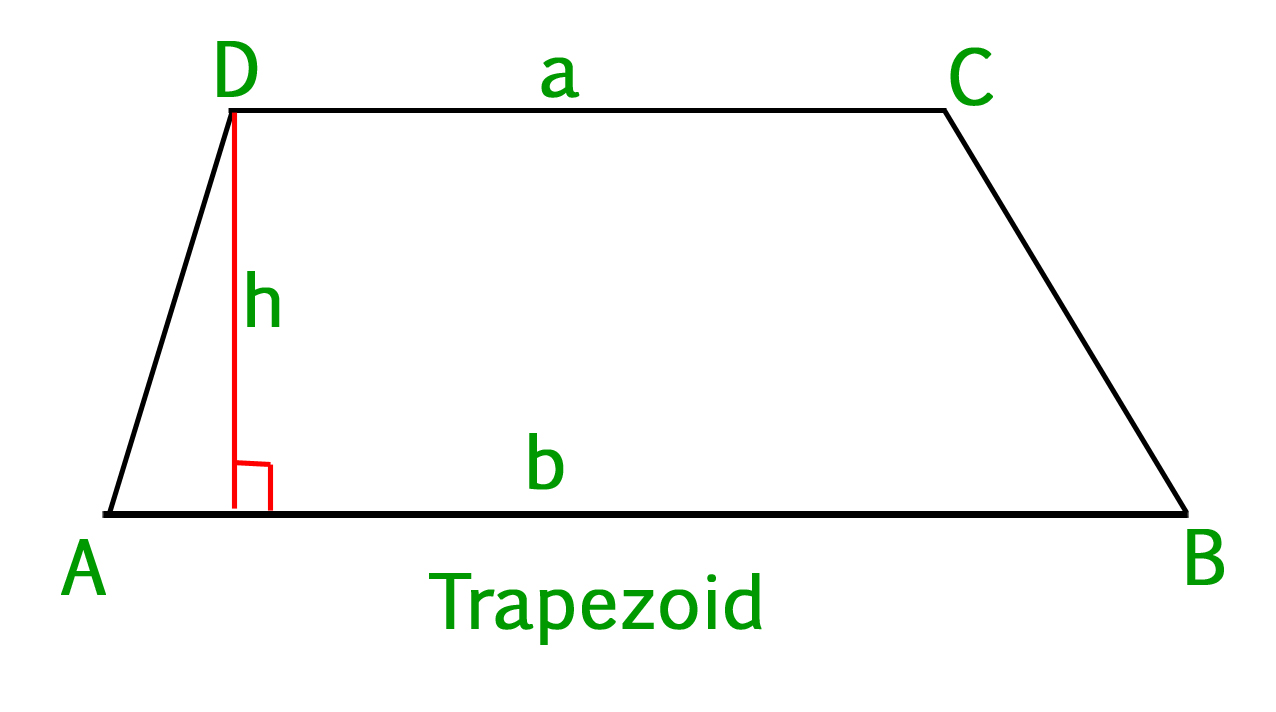Program to find area of a Trapezoid

• Last Updated : 18 Mar, 2021

Definition of Trapezoid :
A Trapezoid is a convex quadrilateral with at least one pair of parallel sides. The parallel sides are called the bases of the trapezoid and the other two sides which are not parallel are referred to as the legs. There can also be two pairs of bases.In the above figure CD || AB, so they form the bases and the other two sides i.e., AD and BC form the legs.
The area of a trapezoid can be found by using this simple formula :a = base
b = base
h = height
Examples :

Input : base1 = 8, base2 = 10, height = 6
Output : Area is: 54.0

Input :base1 = 4, base2 = 20, height = 7
Output :Area is: 84.0

C++

 // C++ program to calculate// area of a trapezoid#includeusing namespace std; // Function for the areadouble Area(int b1, int b2,                    int h){    return ((b1 + b2) / 2) * h;} // Driver Codeint main(){    int base1 = 8, base2 = 10,                height = 6;    double area = Area(base1, base2,                            height);    cout << "Area is: " << area;    return 0;} // This code is contributed by shivanisinghss2110

C

 // CPP program to calculate// area of a trapezoid#include  // Function for the areadouble Area(int b1, int b2,                    int h){    return ((b1 + b2) / 2) * h;} // Driver Codeint main(){    int base1 = 8, base2 = 10,                   height = 6;    double area = Area(base1, base2,                              height);    printf("Area is: %.1lf", area);    return 0;}

Java

 // Java program to calculate// area of a trapezoidimport java.io.*; class GFG{         // Function for the area    static double Area(int b1,                       int b2,                       int h)    {        return ((b1 + b2) / 2) * h;    }     // Driver Code    public static void main (String[] args)    {        int base1 = 8, base2 = 10,                       height = 6;        double area = Area(base1, base2,                                  height);        System.out.println("Area is: " + area);    }}

Python3

 # Python program to calculate# area of a trapezoid # Function for the areadef Area(b1, b2, h):    return ((b1 + b2) / 2) * h # Driver Codebase1 = 8; base2 = 10; height = 6area = Area(base1, base2, height)print("Area is:", area)

C#

 // C# program to calculate// area of a trapezoidusing System; class GFG{         // Function for the area    static double Area(int b1,                       int b2,                       int h)    {        return ((b1 + b2) / 2) * h;    }     // Driver Code    public static void Main ()    {        int base1 = 8, base2 = 10,                       height = 6;        double area = Area(base1, base2,                                  height);        Console.WriteLine("Area is: " + area);    }} // This code is contributed by vt_m



Javascript



Output :

Area is: 54.0

My Personal Notes arrow_drop_up